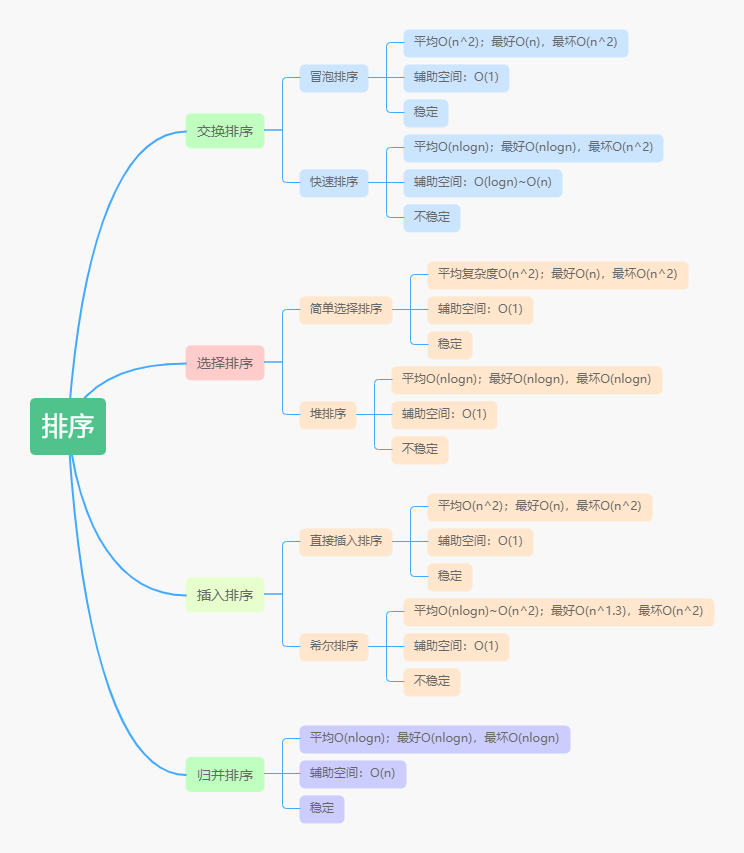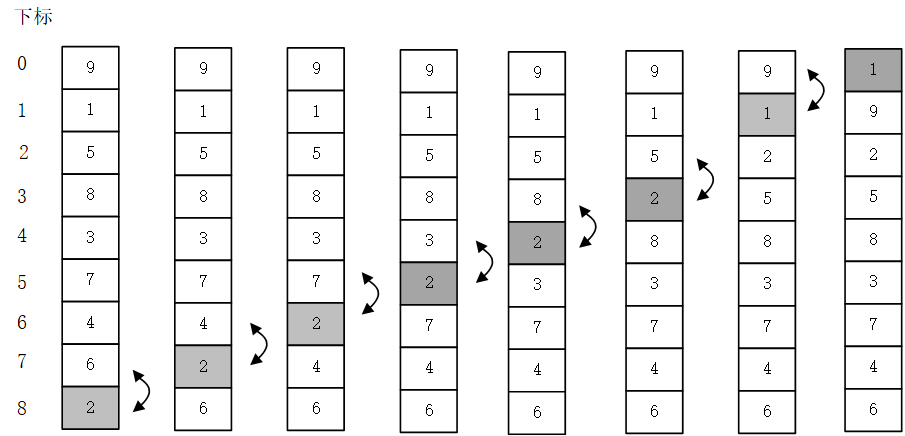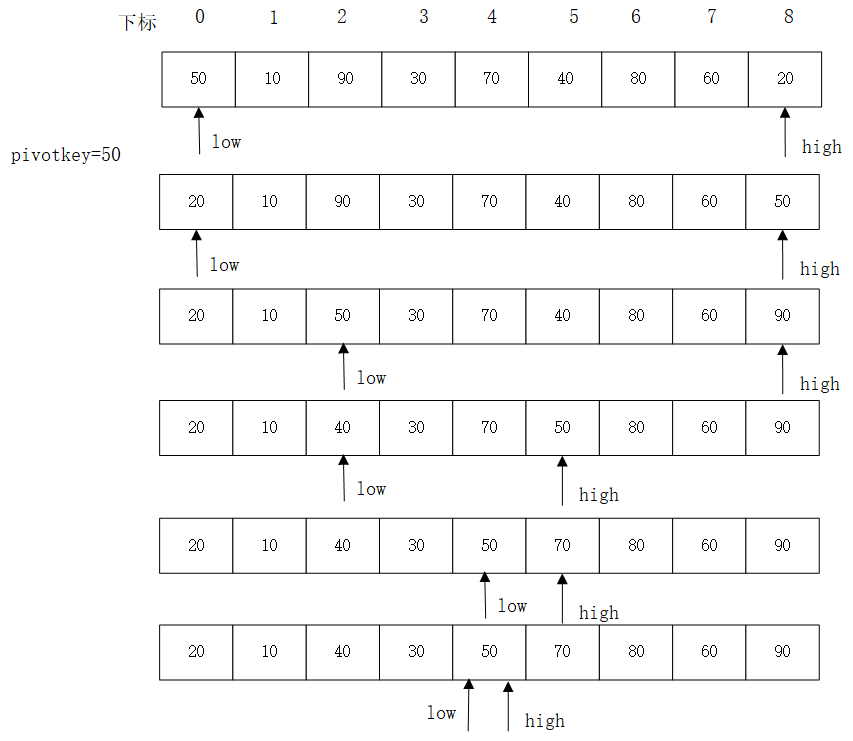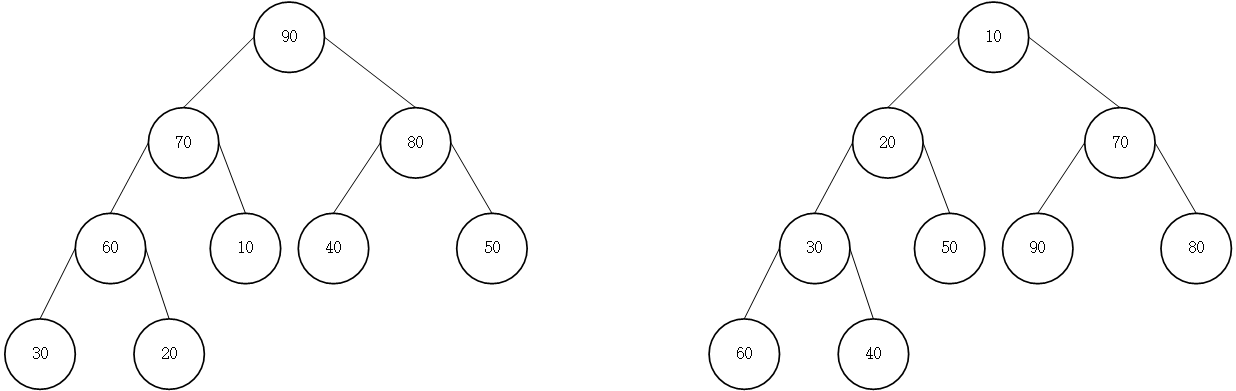# 大話數據結構讀書筆記-排序

## 1.排序的基本概念與分類

### 1.2 內排序與外排序

1. 時間性能

排序算法的時間開銷是衡量其好壞的最重要標志。在內排序中，主要進行兩種操作：比較和移動。高效的排序算法應該具有盡可能少的關鍵字比較次數和盡可能少的移動次數。

2. 輔助空間

評價排序算法的另一個標準是執行算法所需要的輔助存儲空間，即除存放待排序所占用的存儲空間之外，執行算法所需要的其他存儲空間。

3. 算法的復雜性

這里指算法本身的復雜度。? 圖1. 7種內排序算法總覽

## 2.交換排序

### 2.1 冒泡排序

#### 2.1.1 冒泡排序算法

class Sort:
# 冒泡排序
def BubbleSort(self, array):
length = len(array)
i = 0
while i < length-1:
j = length - 1
while j > i:
if array[j-1] > array[j]:
array[j-1], array[j] = array[j], array[j-1]
j -= 1
i += 1
return array#### 2.1.2 冒泡排序優化

    def BubbleSort_2(self, array):
length = len(array)
flag = True
i = 0
while i < length - 1 and flag:
flag = False
j = length - 1
while j > i:
if array[j-1] > array[j]:
array[j-1], array[j] = array[j], array[j-1]
flag = True
j -= 1
i += 1
return array


## 2.2 快速排序

#### 2.2.1 快速排序

    def QuickSort(self, array):
length = len(array)
self.Qsort(array, 0, length-1)
return array

def QSort(self, array, low, high):
if low < high:
pivot = self.Partition(array, low, high)
self.QSort(array, low, pivot-1)
self.QSort(array, pivot+1, high)

def Partition(self, array, low, high):
pivotKey = array[low]
while low < high:
while low < high and array[high] >= pivotKey:
high -= 1
array[low], array[high] = array[high], array[low]
while low < high and array[low] < pivotKey:
low += 1
array[low], array[high] = array[high], array[low]
return low圖3. 快排模擬

#### 2.2.2 快速排序優化

1.優化選取樞軸

def Partition(self, array, low, high):
m = low + (high - low) / 2
if array[low]>array[high]:
array[low], array[high] = array[high], array[low]
if array[m]>array[high]:
array[m], array[high] = array[high], array[m]
if array[low] > array[m]:
array[low], array[m] = array[m], array[low]
pivotKey = array[low]
while low < high:
while low < high and array[high] >= pivotKey:
high -= 1
array[low], array[high] = array[high], array[low]
while low < high and array[low] < pivotKey:
low += 1
array[low], array[high] = array[high], array[low]
return low


2.優化不必要的交換

    def Parition(self, array, low, high):
m = low + (high - low) // 2
print(low, high, m)
if array[low] > array[high]:
array[low], array[high] = array[high], array[low]
if array[m] > array[high]:
array[m], array[high] = array[high], array[m]
if array[low] > array[m]:
array[low], array[m] = array[m], array[low]
pivotKey = array[low]
tmp = pivotKey
while low < high:
while low < high and array[high] >= pivotKey:
high -= 1
array[low] = array[high]
while low < high and array[low] < pivotKey:
low += 1
array[high] = array[low]
array[low] = tmp
return low


3.優化小數組時的排序方案

 	# 設置一個常數， MAX_LENGTH_INSERT_SORT
def QSort(self, array, low, high):
if high-low > MAX_LENGTH_INSERT_SORT:
pivot = self.Parition(array, low, high)
self.QSort(array, low, pivot-1)
self.QSort(array, pivot+1, high)
else:
# 小于等于常數時用直接插入排序
InsertSort(array)


4.優化遞歸操作

    def QSort_1(self, array, low, high):
while low < high:
pivot = self.Parition(array, low, high)
self.QSort_1(array, low, pivot-1)
low = pivot + 1


## 3. 選擇排序

### 3.1 簡單選擇排序

#### 3.1.1 簡單選擇排序算法

    def SimpleSelectionSort(self, array):
length = len(array)
for i in range(length-1):
minv = i
for j in range(i+1, length):
if array[minv] > array[j]: # 較小的值位置賦給minv
minv = j
if minv != i: # minv 不等于 i,則說明找到最小值，交換
array[i], array[minv] = array[minv], array[i]
return array



### 3.2 堆排序

#### 3.2.1 堆圖4 堆

$\begin{cases} k_i \geq k_{2i} \\ k_i \geq k_{2i+1} \end{cases} 或 \begin{cases} k_i \leq k_{2i} \\ k_i \leq k_{2i+1} \end{cases} 1\leq i \leq \lfloor{\frac{n}{2}}\rfloor$

#### 3.2.2 堆排序算法

1. 如何由一個無序序列構建一個堆？
2. 在輸出堆頂元素后，如何調整剩余元素成為一個新的堆？

    def HeapSort(self, array):
length = len(array)
i = length // 2
while i >= 0:
i -= 1
i = length-1
while i > 0:
array[i], array = array, array[i] # 將當前堆頂元素與第i個元素交換，實現
i -= 1
return array

if m == 0: # m == 0 時好跳出循環
return
temp = array[s]
j = 2 * s
while j <= m:
if j < m and array[j] < array[j+1]: # 調整位置s的元素，將其作為它后面元素的堆根
j += 1						    # 結點
if temp > array[j]:
break
array[s] = array[j]
s = j
j = j*2
array[s] = temp



## 4.插入排序

### 4.1 直接插入排序

#### 4.1.1 直接插入排序算法

   def InsertSort(self, array):
length = len(array)
for i in range(1, length):
if array[i] < array[i-1]:
tmp = array[i]
j = i-1
while array[j] > tmp and j >= 0:
array[j+1] = array[j] #
j -= 1
array[j+1] = tmp
return array



### 4.2 希爾排序

#### 4.2.1 希爾排序算法

    def ShellSort(self, array):
length = len(array)
increment = length
while increment > 1:
increment = increment // 3 + 1
for i in range(increment, length): # 相隔 increment進行直接插入排序
if array[i] < array[i-increment]:
temp = array[i]
j = i - increment
while j >= 0 and temp < array[j]:
array[j+increment] = array[j]
j -= increment
array[j+increment] = temp
return array



## 5.歸并排序

#### 5.1 歸并排序算法

 def MergeSort(self, array):
self.MSort(array, 0, len(array))
return array

def MSort(self, array, s, t):
if s == t:
return
else:
m = (s+t) // 2
self.MSort(array, s, m)
self.MSort(array, m+1, t)
self.Merge(array, s, m+1, t)

def Merge(self, SR, s, m, n):

left = []
right = []
for i in range(s, m):
left.append(SR[i])
for i in range(m, n):
right.append(SR[i])
left_size = m - s
right_size = n - m
i = 0
j = 0
k = s
while i<left_size and j < right_size:
if left[i] < right[j]:
SR[k]=left[i]
i+=1
else:
SR[k]=right[j]
j+=1
k+=1
while i<left_size:
SR[k]=left[i]
k+=1
i+=1
while j<right_size:
SR[k]=right[j]
k+=1
j+=1



#### 5.2 歸并排序復雜度分析

posted @ 2020-04-29 15:09  川南煙雨  閱讀(...)  評論(...編輯  收藏